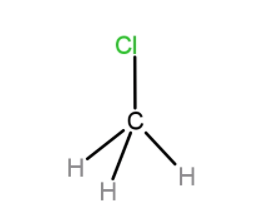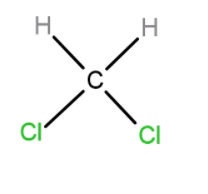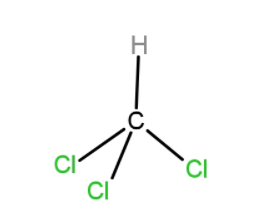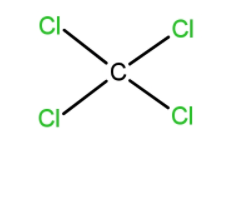Which of the following molecules has the highest dipole moment?A. $C{{H}_{3}}Cl$B. $C{{(H)}_{2}}{{(Cl)}_{2}}$C. $CH{{(Cl)}_{3}}$D. $C{{(Cl)}_{4}}$Verified
76.4k+ views
Hint: To solve this question you should have basic knowledge of dipole moment, structures of given molecules, difference between bond dipole moment and net dipole moment.

Complete answer: A dipole moment arises in any system in which there is a separation of charge. They can arise in ionic bonds as well as in covalent bonds. Dipole moments occur due to the difference in electronegativity between two chemically bonded atoms. The measure of the polarity of a chemical bond between two atoms in a molecule is known as the bond dipole moment of that molecule.
As asked in question among $C{{H}_{3}}Cl$, $C{{(H)}_{2}}{{(Cl)}_{2}}$, $CH{{(Cl)}_{3}}$ and $C{{(Cl)}_{4}}$ which molecule has highest dipole moment.
A. $C{{H}_{3}}Cl$In the case of $C{{H}_{3}}Cl$, the dipole moment between C and H bond is 0. Hence, only the Cl group contributes to the dipole moment of the molecule.
B. $C{{(H)}_{2}}{{(Cl)}_{2}}$In the case of $C{{(H)}_{2}}{{(Cl)}_{2}}$, both Cl groups contribute to the dipole moment so it has the highest dipole moment amongst all.
C. $CH{{(Cl)}_{3}}$In the case of $CH{{(Cl)}_{3}}$, one of the Cl cancels the dipole moment of the opposite Cl atom, so net dipole moment is just due to one Cl.
D. $C{{(Cl)}_{4}}$We know that for symmetrical compounds the dipole moment is always zero, as $C{{(Cl)}_{4}}$there is a symmetrical compound so its dipole moment will be zero. Hence, $C{{(Cl)}_{4}}$ is non-polar. $C{{(Cl)}_{4}}$ is a symmetrical compound so its dipole moment will be zero. Hence, $C{{(Cl)}_{4}}$ is non-polar.
Hence, $C{{(H)}_{2}}{{(Cl)}_{2}}$ has the highest dipole moment amongst the given options.

Hence, B is the right answer.

Note:These molecules have tetrahedral geometry due to sp3 hybridization of carbon atoms.
We know that for symmetrical compounds the dipole moment is always zero. Hence, they are non-polar.
In the case of a single bond in a polyatomic molecule its dipole moment is known as the bond dipole moment and this dipole moment is different from the dipole moment of the whole molecule.